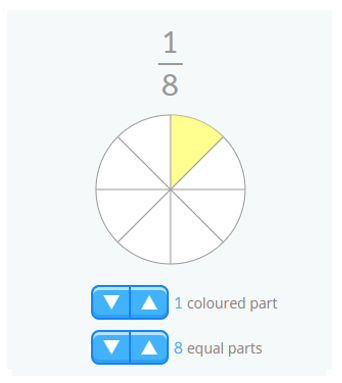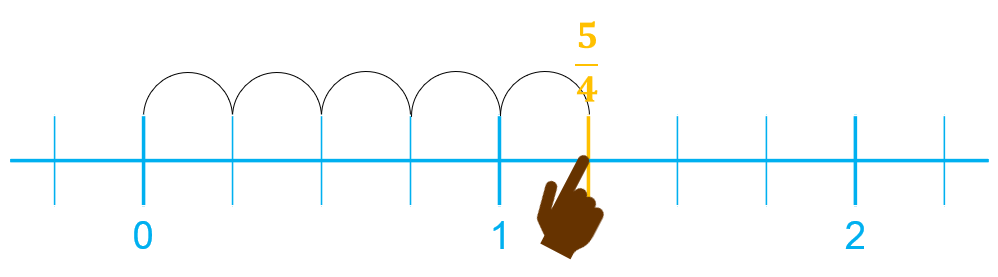Smartick is an online platform for children to master math in only 15 minutes a day

Mar05

# Understanding Fractions: “If the Whole Is Made of 8 Parts, How Can I Have 11?”

We’ve written plenty about fractions in this blog. Recently, one of our readers asked us to explain the content of this post on classification of fractions in more detail. His comment was: “I’m sorry but I don’t understand improper fractions… I think it’s impossible to have 11 parts of something that’s divided into 8”.

When we learn and work with fractions (or any other subject of mathematics) we must keep something in mind: mathematics is a very specific discipline. These posts will give you an example of what we mean:

Therefore, many aspects of mathematics can be understood in different ways. That’s the case with fractions. Our reader only understood them as the relation of a part to a whole (“it’s impossible to have 11 parts of something that’s divided into 8”), but fractions can be understood in other ways.

Here we explain three different ways to understand a fraction:

### 1. Fractions as a part-whole relation:

This is one of the first applications of fractions we learn. It’s a way to express a relation between the part we have and the whole we’ve taken it from.

For example, I took an eighth of cake: This means that of all the cake, which was divided into 8 equal pieces, I only took one piece.### 2. Fractions as a relation between different elements:

On the other hand, we can use a fraction to demonstrate the relation between one object and another, where the relation is constant. For example, the relation that exists between a hand and its fingers is 1/5, because there are 5 fingers for each hand.

###3. Fractions as numbers that we can represent as points on the number line:

However, fractions are also numbers that we can represent on the number line. This is different to the previous idea of a “whole” or there being only one unit. The unit will be divided into as many parts as indicated by the denominator as usual, and the numerator will indicate a specific point on the number line.

We’re going to take a look at two examples:

• A proper fraction is smaller than the unit:

• An improper fraction is greater than the unit:This is why sometimes we can take eleven eighths of a cake, because the denominator is indicating the number of equal parts that the unit is divided into, not the “whole” or total amount of cake we have… problem solved!If you want to keep learning different ways to use fractions, log into Smartick and try it free.